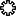# The Inverse and Determinants of 2x2 and 3x3 Matrices

For those people who need instant formulas!
The general way to calculate the inverse of any square matrix, is to append a unity matrix after the matrix (i.e. [A | I]), and then do a row reduction until the matrix is of the form [I | B], and then B is the inverse of A. There is also a general formula based on matrix conjugates and the determinant.
In the following, DET is the determinant of the matrices at the left-hand side.

## The inverse of a 2x2 matrix:

```| a11 a12 |-1             |  a22 -a12 |
| a21 a22 |    =  1/DET * | -a21  a11 |

with DET  =  a11a22-a12a21
```

## The inverse of a 3x3 matrix:

```| a11 a12 a13 |-1
| a21 a22 a23 |    =  1/DET * A
| a31 a32 a33 |

with A  =

|  a33a22-a32a23  -(a33a12-a32a13)   a23a12-a22a13 |
|-(a33a21-a31a23)   a33a11-a31a13  -(a23a11-a21a13)|
|  a32a21-a31a22  -(a32a11-a31a12)   a22a11-a21a12 |

and DET  =  a11(a33a22-a32a23)
- a21(a33a12-a32a13)
+ a31(a23a12-a22a13)
```

Alexander Thomaswww.dr-lex.be